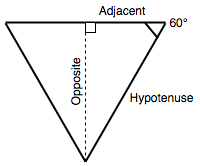# Math

The `Math` namespace object contains static properties and methods for mathematical constants and functions.

`Math` works with the `Number` type. It doesn't work with `BigInt`.

## Description

Unlike most global objects, `Math` is not a constructor. You cannot use it with the `new` operator or invoke the `Atomics` object as a function. All properties and methods of `Math` are static.

Note: Many `Math` functions have a precision that's implementation-dependent.

This means that different browsers can give a different result. Even the same JavaScript engine on a different OS or architecture can give different results!

## Static properties

`Math.E`

Euler's number and the base of natural logarithms; approximately `2.718`.

`Math.LN10`

Natural logarithm of `10`; approximately `2.303`.

`Math.LN2`

Natural logarithm of `2`; approximately `0.693`.

`Math.LOG10E`

Base-10 logarithm of `E`; approximately `0.434`.

`Math.LOG2E`

Base-2 logarithm of `E`; approximately `1.443`.

`Math.PI`

Ratio of a circle's circumference to its diameter; approximately `3.14159`.

`Math.SQRT1_2`

Square root of ½; approximately `0.707`.

`Math.SQRT2`

Square root of `2`; approximately `1.414`.

`Math[@@toStringTag]`

The initial value of the `@@toStringTag` property is the string `"Math"`. This property is used in `Object.prototype.toString()`.

## Static methods

`Math.abs()`

Returns the absolute value of `x`.

`Math.acos()`

Returns the arccosine of `x`.

`Math.acosh()`

Returns the hyperbolic arccosine of `x`.

`Math.asin()`

Returns the arcsine of `x`.

`Math.asinh()`

Returns the hyperbolic arcsine of a number.

`Math.atan()`

Returns the arctangent of `x`.

`Math.atan2()`

Returns the arctangent of the quotient of its arguments.

`Math.atanh()`

Returns the hyperbolic arctangent of `x`.

`Math.cbrt()`

Returns the cube root of `x`.

`Math.ceil()`

Returns the smallest integer greater than or equal to `x`.

`Math.clz32()`

Returns the number of leading zero bits of the 32-bit integer `x`.

`Math.cos()`

Returns the cosine of `x`.

`Math.cosh()`

Returns the hyperbolic cosine of `x`.

`Math.exp()`

Returns ex, where x is the argument, and e is Euler's number (`2.718`…, the base of the natural logarithm).

`Math.expm1()`

Returns subtracting `1` from `exp(x)`.

`Math.floor()`

Returns the largest integer less than or equal to `x`.

`Math.fround()`

Returns the nearest single precision float representation of `x`.

`Math.hypot()`

Returns the square root of the sum of squares of its arguments.

`Math.imul()`

Returns the result of the 32-bit integer multiplication of `x` and `y`.

`Math.log()`

Returns the natural logarithm (㏒e; also, ㏑) of `x`.

`Math.log10()`

Returns the base-10 logarithm of `x`.

`Math.log1p()`

Returns the natural logarithm (㏒e; also ㏑) of `1 + x` for the number `x`.

`Math.log2()`

Returns the base-2 logarithm of `x`.

`Math.max()`

Returns the largest of zero or more numbers.

`Math.min()`

Returns the smallest of zero or more numbers.

`Math.pow()`

Returns base `x` to the exponent power `y` (that is, `x``y`).

`Math.random()`

Returns a pseudo-random number between `0` and `1`.

`Math.round()`

Returns the value of the number `x` rounded to the nearest integer.

`Math.sign()`

Returns the sign of the `x`, indicating whether `x` is positive, negative, or zero.

`Math.sin()`

Returns the sine of `x`.

`Math.sinh()`

Returns the hyperbolic sine of `x`.

`Math.sqrt()`

Returns the positive square root of `x`.

`Math.tan()`

Returns the tangent of `x`.

`Math.tanh()`

Returns the hyperbolic tangent of `x`.

`Math.trunc()`

Returns the integer portion of `x`, removing any fractional digits.

## Examples

### Converting between degrees and radians

The trigonometric functions `sin()`, `cos()`, `tan()`, `asin()`, `acos()`, `atan()`, and `atan2()` expect (and return) angles in radians.

Since humans tend to think in degrees, and some functions (such as CSS transforms) can accept degrees, it is a good idea to keep functions handy that convert between the two:

``````function degToRad(degrees) {
return degrees * (Math.PI / 180);
}

return rad / (Math.PI / 180);
}
``````

### Calculating the height of an equilateral triangle

If we want to calculate the height of an equilateral triangle, and we know its side length is 100, we can use the formulae length of the adjacent multiplied by the tangent of the angle is equal to the opposite.In JavaScript, we can do this with the following:

``````50 * Math.tan(degToRad(60));
``````

We use our `degToRad()` function to convert 60 degrees to radians, as `Math.tan()` expects an input value in radians.

### Returning a random integer between two bounds

This can be achieved with a combination of `Math.random()` and `Math.floor()`:

``````function random(min, max) {
const num = Math.floor(Math.random() * (max - min + 1)) + min;
return num;
}

random(1, 10);
``````

## Specifications

Specification
ECMAScript Language Specification
# sec-math-object

## Browser compatibility

BCD tables only load in the browser